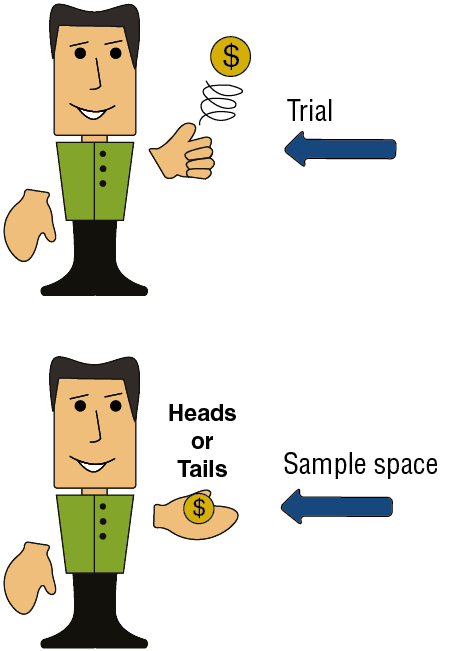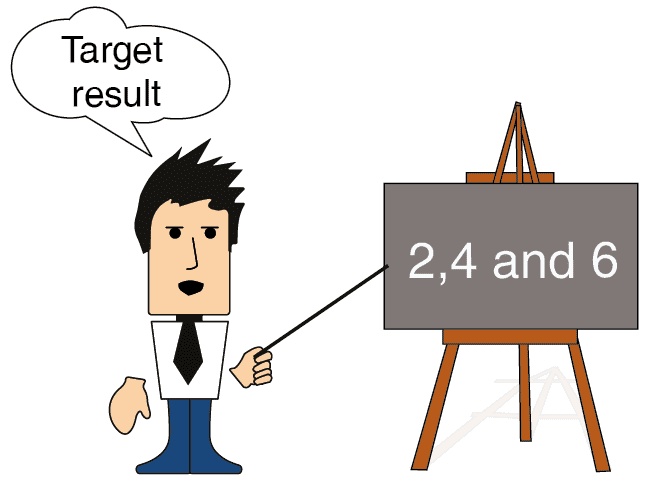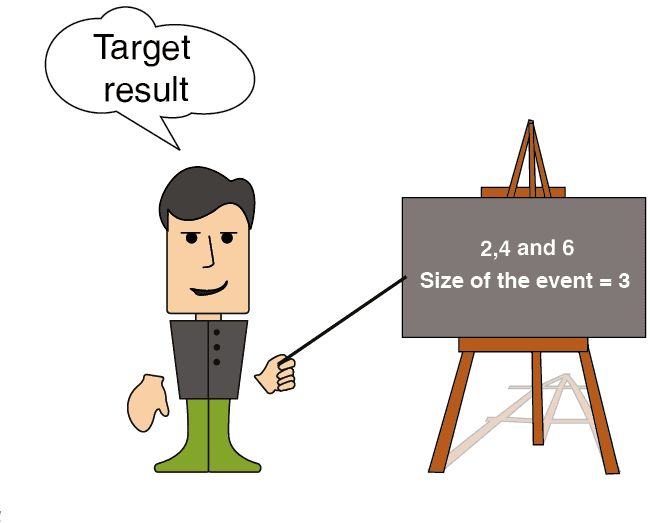# Statistical Terms Explained

Before we progress to the second section of the course on probability, however, we will learn several more terms that will help us to understand the subject. The terms are as follows:

• Trial

• The sample space

• Size of the sample space

• Event

• Size of the event

## Trial

The term trial refers to any action, the results of which are not known in advance.
Tossing a coin is a trial since the result can be “heads” or “tails”.
Throwing the dice can result in any whole number between 1 and 6.## The sample space

The sample space refers to all of the possible results of a trial.
For example, when tossing a coin, the sample space is “heads” and “tails”, because these represent the only possible results of the trial.
When throwing the dice, the sample space is 1, 2, 3, 4, 5, and 6.
Size of the Sample Space
The number of different values in the sample space is called the size of the sample space.
Examples:

• The size of the sample space when tossing a coin is 2 (”heads” and “tails”).

• The size of the sample space when throwing a dice is 6 (1, 2, 3, 4, 5, and 6).

• The size of the sample space when drawing a playing card without jokers from the deck is 52.An Example to Summarize

We will practice using the new terms in another example:

Tossing two coins simultaneously.

The trial: A single tossing of two coins.

The sample space: What are the possible results from the trial? The answer is as follows:

• HH (”heads” on the first coin and “heads” on the second coin).

• HT (”heads” on the first coin and “tails” on the second coin).

• TH (”tails” on the first coin and “heads” on the second coin).

• TT (”tails” on the first coin and “tails” on the second coin).

The size of the sample space is 4 (because there are four possibilities in the sample).An event occurs when a result is obtained during a trial that we have “marked” in advance (i.e., a target result). We will consider the coin toss. If we have marked in advance the results 2 and 3 (out of the six total possible results), then, if the result obtained when throwing one of the dice is 2 or 3, then we “celebrate” an event since we predicted that the event would occur. If one of the other events (1, 4, 5, or 6) results, then we say that the predicted event did not occur.

Another example: If, when throwing the dice, we define the “target result” as an even number, i.e. we mark in advance the results 2, 4, and 6, then if these results are obtained, we “celebrate” an event.

In any other case (1, 3, or 5), we say that the event did not occur.

The size of the event:

The size of the event reflects the number of different values relative to an event that we have defined.

Examples:

The size of the event (i.e., an even number is obtained when throwing one of the dice) is 3 since the event has three values: 2, 4, and 6.

The size of the event when a king is obtained while drawing a card from the pack is 4 since the event has 4 values: i.e., one king of each suit .